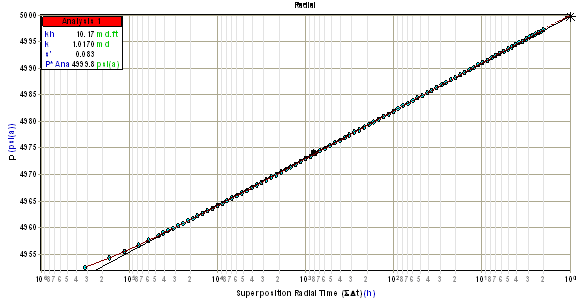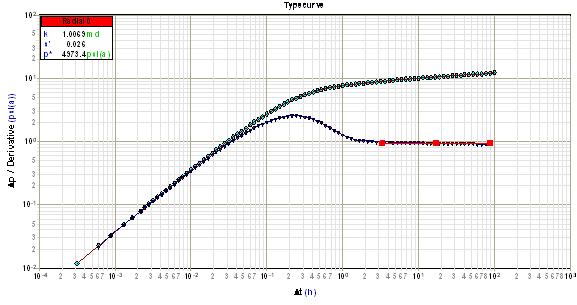Subtopics:

Radial flow is a flow regime that occurs in the middle time (infinite-acting) region, before the occurrence of boundary effects. The purpose of analyzing radial flow data is to determine permeability (k), and apparent or total skin (s').

## Constant Rate Solution

For oil and water:For gas:Radial flow data will form a straight line when placed on a semi-log plot of pressure versus a log time function (p vs. log(time function)) for a buildup as shown below.### Permeability

The slope (m) of the p vs. log(time function) line is used to calculate permeability as follows:

For oil and water:For gas:### Skin

#### Drawdown Tests

Permeability along with the slope (m) and position of the line is used to calculate apparent or total skin (s'). The equation for total skin (s') is found by rearranging the radial flow equation as follows:

For oil and water:For gas:The values of pwf and t can be taken from any point on the semi-log straight line (not a measured data point).

#### Buildup Tests

For a buildup test, total skin (s') cannot be determined from shut-in pressure alone. It can still be found however, by making use of the information provided by any flowing pressure point.  By convention, the final flowing pressure (pwfo) is used.Any pressure off the semi-log line (not the measured data) can be used, corresponding to any specified shut-in time. In most texts the pressure at 1 hour is used.  In the software, the extrapolated buildup pressure (p*) at infinite shut-in time (t = 1.0) is used in the total skin (s') calculation. Using this convention, the equation used for calculating total skin (s') from a buildup test is as follows:

For oil and water:For gas:## Summary of Equations for Radial Flow (Field Units)

Flow Period Permeability (md) Apparent Skin

Oil

DrawdownBuildupGas

DrawdownBuildup## Other Required Equations (Gas Equations, Field Units)

 Radius of InvestigationPressure Drop Due to Skin## Derivative

The signature of radial flow data on a derivative plot is a horizontal straight line. The position of this line is used to calculate permeability (k), and apparent or total skin (s'). Note that an increase in the vertical position of the horizontal straight line corresponds to a decrease in permeability.

Starting with the constant rate solution (see above) the derivative with respect to the logarithm of time is:

For oil and water:For gas:At long flow times, this result is linear with time, and as a result, the derivative of radial flow data falls on a horizontal straight line with a slope equal to 0 on a log Dp versus log Dt plot as shown below.  Note that for shorter flow times, radial equivalent time should be used to maintain a horizontal slope.### Permeability

The value of the derivative of radial flow data corresponds to the vertical position of the horizontal straight line and is used to calculate permeability (k) as follows:

For oil and water:For gas:### Skin

An equation for calculating apparent or total skin (s') is found by substituting the above equation for permeability (k) into the constant rate solution.

## Summary of Equations for Radial Flow Derivative Analysis (Field Units)

Flow Period Permeability (md) Apparent Skin

Oil

DrawdownBuildupGas

DrawdownBuildup